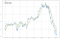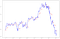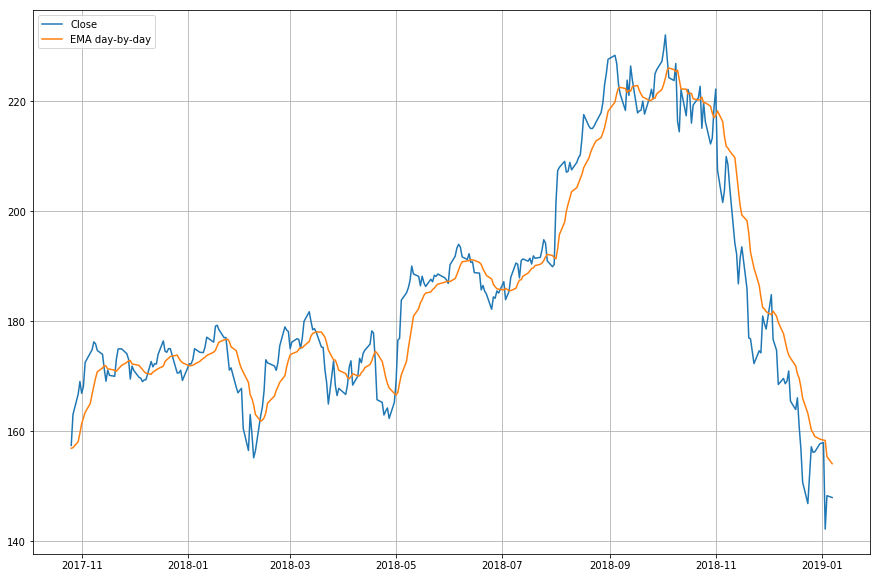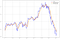# Predicting stock prices with LSTM

In this article we will use Neural Network, specifically the LSTM model, to predict the behaviour of a Time-series data. The problem to be solved is the classic stock market prediction. All data used and code are available in .

Although this is indeed an old problem, it remains unsolved until today. The truth is quite simple: the stock price is determined by several factors, and its historical price is just a small fraction of it. As a result, predicting the price behaviour is a really difficult problem, unless you are a .

# Summary

First, I will introduce the dataset with some data visualisation. Then, I will briefly discuss how difficult it is to predict the stock market behaviour by using the moving average algorithm and showing its limitations. Next, a short introduction to the concept os Recurrent Neural Networks and LSTM, followed by a LSTM example of predicting the stock price for a single company. Finally, I will show the LSTM used to predict the price of four companies at the same time and compare the results to see if the prediction improves as we use more companies at the same time.

# Data visualisation

The dataset was downloaded from in CSV. It has the stock price of four companies in the period between 01/08/2010 and 01/07/2019. We will refer to them as company A, B, C and D.

The basic step is to open the CSV file using Pandas. In a first look at the data, we have:

`df_A = pd.read_csv(‘data/company_A.csv’)df_A[‘Date’] = pd.to_datetime(df_A[‘Date’])df_A.tail()`
`plt.figure(figsize = (15,10))plt.plot(df_A['Date'], df_A['Close'], label='Company A')plt.plot(df_B['Date'], df_B['Close'], label='Company B')plt.plot(df_C['Date'], df_C['Close'], label='Company C')plt.plot(df_D['Date'], df_D['Close'], label='Company D')plt.legend(loc='best')plt.show()`

# Moving Average

A classic algorithm used in this problem is the Moving Average (MA). It consists in calculating the average of the `m` past observed days and use this result as the next day prediction. To demonstrate, here is an example of a moving average using `m` as 10 and 20 days on company's A closing price.

`df['MA_window_10'] = df['Close'].rolling(10).mean().shift() #shift so the day we want to predict won't be useddf['MA_window_20'] = df['Close'].rolling(20).mean().shift()`One step-ahed prediction of the closing value of company A using Moving Average.

When we try to forecast the closing price 10 days ahead using Moving Average, the results are as follows:10 days closing price prediction of company A using Moving Average

Notice that each red line represents a 10 day prediction based on the 10 past days. For this reason, the red line is discontinuous.

Using a slightly fancier algorithm, the Exponential Moving Average (EMA), we achieve some small improvement:One step-ahed prediction of the closing value of company A using Exponential Moving Average.

Contrasting the MA and EMA:Comparison of one step-ahed prediction of the closing value of company A using MA and EMA

This approach is simplistic. What we really want is to predict `n` days ahead to see stock the future behave, and both MA and EMA fail in this task.

# Recurrent Neural Network (RNN)

To understand an LSTM Network, we need to understand a Recurrent Neural Network first. This kind of network is used to recognise patterns when past results have influence on the present result. An example of RNN usage is the time-series functions, in which the data order is extremely important.

In this network architecture, the neuron uses as input not only the regular input (the previous layer output), but also its previous state.

It is important to notice that `H` represents the neuron state. Therefore, when in state `H_1`, the neuron uses as input the parameter `X_1` and `H_0` (its previous state). The main problem of this model is the memory loss. The network older states are fast forgotten. In sequences where we need to remember beyond the immediate past, RNNs fail to remember.

# LSTM Network

An LSTM Network has its origin in a RNN. But it can solve the memory loss by changing the neuron architecture.

The new neuron has 3 gates, each with a different goal. The gates are:

• Input Gate
• Output Gate
• Forget Gate

An LSTM Neuron still receives as input its previous state:

# LSTM to predict a single company

At last, let's use an LSTM to predict the behaviour of company A alone.

But first, consider the following parameters. We want to predict the `n` days ahead (`foward_days`) having as input the `m` past observed days (`look_back`). So, if we have an input of `m` past days, the network output will be the prediction for the `n` next days. We will split the data in Train and Test. The test will be composed of `k` periods (`num_periods`), in which every period is a series of `n` days prediction.

`look_back = 40forward_days = 10num_periods = 20`

Now, we open the CSV file with Pandas and keep only the columns we will use, that are the date and the closing price. The initial closing price graphic for company A is:

`plt.figure(figsize = (15,10))plt.plot(df)plt.show()`

In sequence, we scale the input, split the data in Train/Validation and Test and format it to feed the model. All the detailed process can be found at my GitHub.

Now, we build and train the model.

`NUM_NEURONS_FirstLayer = 128NUM_NEURONS_SecondLayer = 64EPOCHS = 220#Build the modelmodel = Sequential()model.add(LSTM(NUM_NEURONS_FirstLayer,input_shape=(look_back,1), return_sequences=True))model.add(LSTM(NUM_NEURONS_SecondLayer,input_shape=(NUM_NEURONS_FirstLayer,1)))model.add(Dense(foward_days))model.compile(loss='mean_squared_error', optimizer='adam')history = model.fit(X_train,y_train,epochs=EPOCHS,validation_data=(X_validate,y_validate),shuffle=True,batch_size=2, verbose=2)`

The result obtained is:

Looking the test set closer:

Notice that each red line represents a 10 days prediction (`forward_days`) based on the 40 past days (`look_back`). There are 20 red lines because we choose to test on 20 periods (`num_periods`). Thats why the prediction line in red is discontinuous.

By repeating the same process for all companies, the best result on the test set was the prediction for company C:

Even though this was the best model, the results are far from great. The reasons that may have caused this result are many. Some of then may be:

• Only the historical data of closing prices is not enough to predict the stock price behaviour
• The model could be improved

# LSTM to predict four companies

Finally, we will use the LSTM model to predict the behaviour of all four companies together, A, B, C and D, and contrast with the single LSTM company results. The goal is to analyse if using the data from several companies can improve the individual prediction of every company.

It is important to point out that all four CSVs have the same dates. This way, the network won't be fed with future information from a company to predict the value of another company.

Initially, the data is:

After the data normalisation and formatting to feed the model, the model was trained:

`NUM_NEURONS_FirstLayer = 100NUM_NEURONS_SecondLayer = 50EPOCHS = 200#Build the modelmodel = Sequential()model.add(LSTM(NUM_NEURONS_FirstLayer,input_shape=(look_back,num_companies), return_sequences=True))model.add(LSTM(NUM_NEURONS_SecondLayer,input_shape=(NUM_NEURONS_FirstLayer,1)))model.add(Dense(foward_days * num_companies))model.compile(loss='mean_squared_error', optimizer='adam')history = model.fit(X_train,y_train,epochs=EPOCHS,validation_data=(X_validate,y_validate),shuffle=True,batch_size=1, verbose=2)`

The result was:

Looking the test set closer:

Time to contrast the results. The results of a the single company LSTM is shown on the left, and the result of the four company LSTM on the right. The first line shows the prediction in the test set, and the second on all the data set.

Company A

Company B

Company C

Company D

# Conclusion

It is not possible to predict the stock market behaviour using only its historical price. The LSTM prediction is far from acceptable. Even when using the historical price of several companies, the prediction got worse.